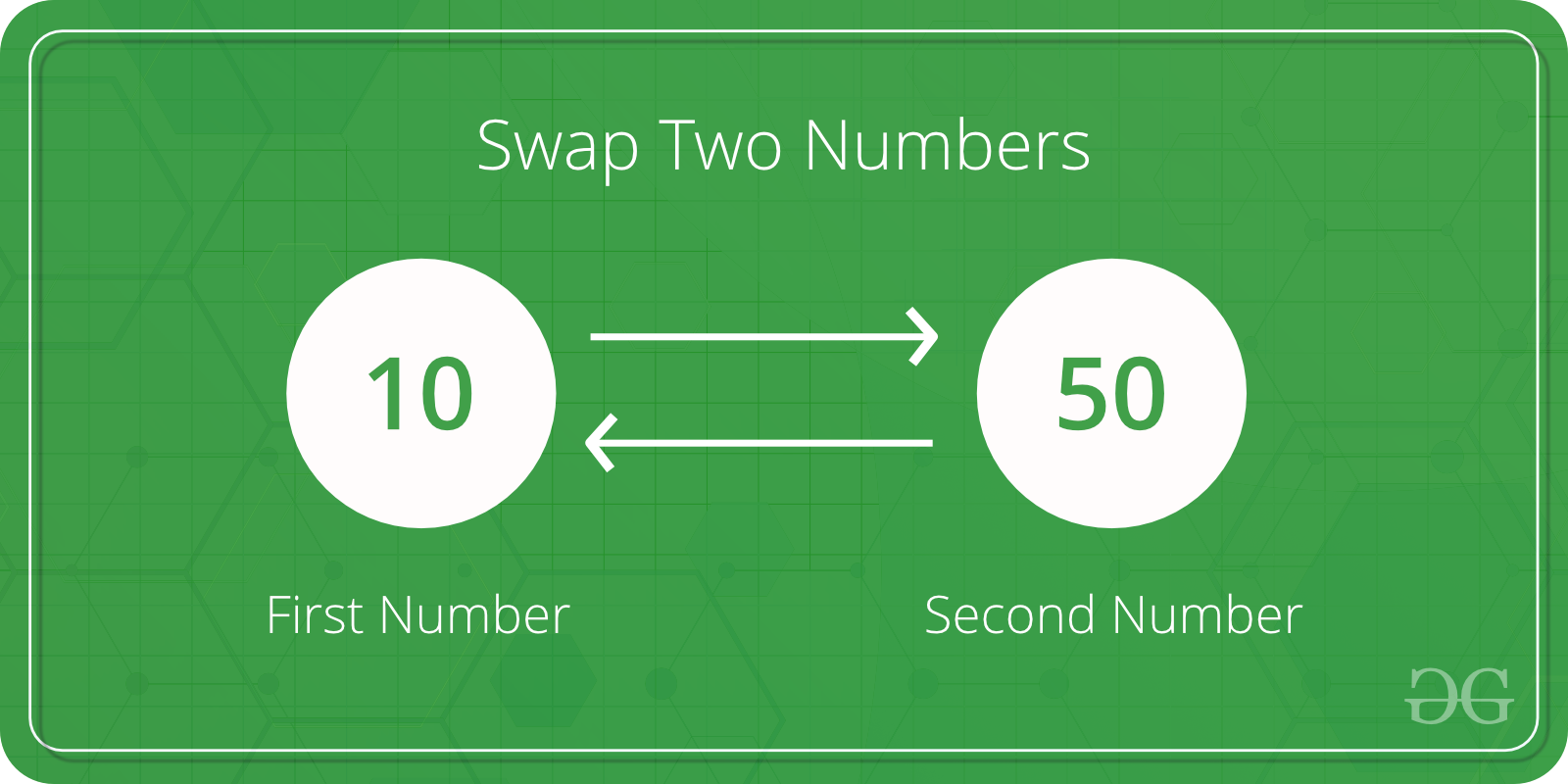# Java Program to Swap two Variables

• Difficulty Level : Basic
• Last Updated : 08 Jun, 2021

Given two numbers x and y, we need to swap their values
Examples:

```Input : x = 10, y = 20;
Output : x = 20, y = 10

Input : x = 200, y = 100
Output : x = 100, y = 200```Below are the simple steps we follow:
1) Assign x to a temp variable : temp = x
2) Assign y to x : x = y
3) Assign temp to y : y = temp
Let us understand with an example.

x = 100, y = 200
After line 1: temp = x
temp = 100
After line 2: x = y
x = 200
After line 3 : y = temp
y = 100

## Java

 `// Java program to swap two variables``public` `class` `GfG {` `    ``public` `static` `void` `main(String[] args)``    ``{` `        ``int` `x = ``100``, y = ``200``;` `        ``System.out.println(``"Before Swap"``);``        ``System.out.println(``"x = "` `+ x);``        ``System.out.println(``"y = "` `+ y);` `        ``int` `temp = x;``        ``x = y;``        ``y = temp;` `        ``System.out.println(``"After swap"``);``        ``System.out.println(``"x = "` `+ x);``        ``System.out.println(``"y = "` `+ y);``    ``}``}`

Output:

```Before Swap
x = 100
y = 200
After swap
x = 200
y = 100```

My Personal Notes arrow_drop_up